Lompat ke konten Lompat ke sidebar Lompat ke footer

# Simulation of wireless power transfer (CHAPTER IV)

### 4.1 Simulation

Figure 4.1 below shows that the transmitter circuit has succeeded in sending electrical energy to the receiving system with a maximum distance of 20 cm using an LED load. After that, the measurement of the V and I quantities will be carried out to find out how much power can be sent from the sender to the receiver if the power transfer distance from the sender to the receiver is changed from 0 to 2 cm, 0 to 4 cm, 0 to 6 cm, and so on. up from 0-20 cm where the results of this measurement will later provide information about the amount of power and efficiency of the WPT system itself. In addition, the author will also test with an oscilloscope to see if the waveforms that can be generated from the sending and receiving systems are as planned or not, which in turn will provide information about the frequency generated by the sending circuit.Figure 4.1 Wireless power simulation

The values ​​of the electrical quantities (V, I) from the measurement and analysis can be seen in the following sub-chapter.

The measurement results of this thesis are basically based on 2 different conditions, namely the condition of the receiving coil winding with an air core (air core) and the condition of the receiving coil winding with an iron sand core (iron sand core). different. However, in this post only the condition of the receiving coil will be shown without the iron sand core. If you want the full version, you can download it from the link above. The results can be seen in Table 4.1 below.Table 4.1 Frequency Variations on the Sender Side

### 4.2 Measurement Without Core (Air Core)

The following measurement results are sorted from the highest frequency to the lowest frequency. Namely from 976 KHz to 651 KHz.

#### 4.3.1 Result of Measurement and Analysis of Wireless Power Transmission System of Air Core Sender and Receiver Coil With 976 KHz Frequency

Figure 4.2 below is a transmitter circuit (oscillator) and a receiver circuit with a resonant frequency of 976 KHz (at the sender) and 944.7 KHz (at the receiver). The frequency is obtained through measurement results with a digital frequency meter, where the shape of the signal (both at the sender and at the receiver) is obtained after going through an oscilloscope test to determine whether the resulting signal is sinusoidal, saw, or square signal. In addition, testing with this oscilloscope also aims to determine whether the frequency shown on the digital meter frequency measuring instrument is the same or at least close to that shown on the oscilloscope. In this circuit, to obtain a frequency value of 976 KHz on the sending side, the capacitors C2 and C3 are in the close/on position. As for the receiving side, it only consists of components L and C which are then paralleled with the load. Where, the value of the receiving frequency does not have to be the same as the sending frequency. The most important thing is that the resonant frequency requirements are met.Figure 4.2 Sender Circuit (Oscillator) 976 KHz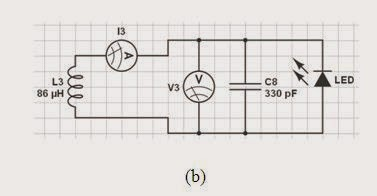Figure 4.2 Receiver Circuit 944.7 KHz

After testing using an oscilloscope, the signal form on the sending and receiving sides is obtained as shown in Figure 4.3 below.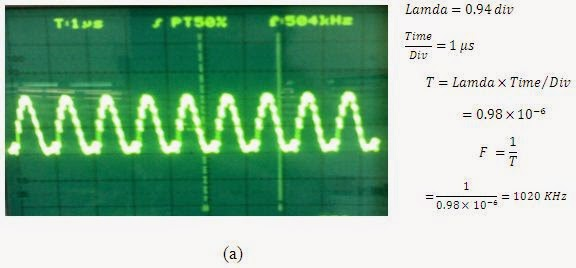Figure 4.3 The form of the sending signal (Oscillator) 976 KHzFigure 4.3 Receiver signal form 944 KHz

In the picture above, it is evident that the signal generated by the sender and captured at the receiver is sinusoidal, and based on the results of the frequency calculation above, it is seen that the frequency value listed on the oscilloscope is slightly different from that produced by a digital frequency meter. This is because the oscilloscope tends to be more precise than the digital frequency meter used by the author. For frequency measurements in the next circuit, it is done using a digital frequency meter on the grounds that it is faster to obtain the desired frequency value.

The current and voltage values after measurement are as follows, where the power value (both source, sender and receiver) is obtained through the results of calculations based on Eq. previous chapter.

#### 4.3.2 Result of Measurement and Analysis of Wireless Power Transmission System of Air Core Receiver Coil With 800 KHz Frequency

To obtain a frequency value of 800 KHz, capacitors C2-C4 in the circuit Figure below are in the on condition (switch close). For more details can be seen in the following image. While on the receiving side, the provisions are in accordance with those described in the previous sub-chapter.Figure 4.4 : (a) Transmitter circuit 800 KHz (b) Receiver signal form 768KHz

The current and voltage values after measurement are as follows, where the power and efficiency values are obtained through the results of calculations as described in the previous sub-chapter.Table 4.3 Measurement results of 800 kHz . Frequency Oscillator

To obtain a frequency value of 780 KHz, the capacitor C2-C5 in the circuit Figure below is in the on condition (switch close). For more details can be seen in the following image. While on the receiving side, the provisions are in accordance with those described in the previous sub-chapter.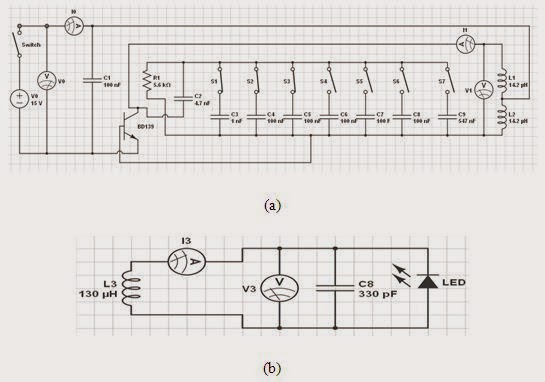Gambar 4.5 : (a) Transmitter of circuit 780 KHz (b) Receiver signal format 768 KHz

The magnitude of the current and voltage values after measurements are made is as follows:Table 4.4 Oscillator Frequency 781 KHz . measurement results

#### 4.3.4 Result of Measurement and Analysis of Wireless Power Transmission System of Air Core Receiver Coil With Frequency 774 KHz

To obtain a frequency value of 774 KHz, the capacitors C2-C6 in the circuit Figure below are in the on condition (switch close). For more details can be seen in the following image. While on the receiving side, the provisions are in accordance with those described in the previous sub-chapter.Figure 4.6 : (a) Transmitter circuit 774 KHz (b) Receiver signal form 768KHz

The magnitude of the current and voltage values after measurement is as follows.Table 4.5 Measurement Results of 774 KHz Oscillator Frequency

#### 4.3.5 Result of Measurement and Analysis of Wireless Power Transmission System of Air Core Receiver Coil With Frequency 725 KHz

To obtain a frequency value of 725 KHz, capacitors C2-C7 in the circuit Figure below are in the on condition (switch close). For more details can be seen in the following image. While on the receiving side, the provisions are in accordance with those described in the previous sub-chapter.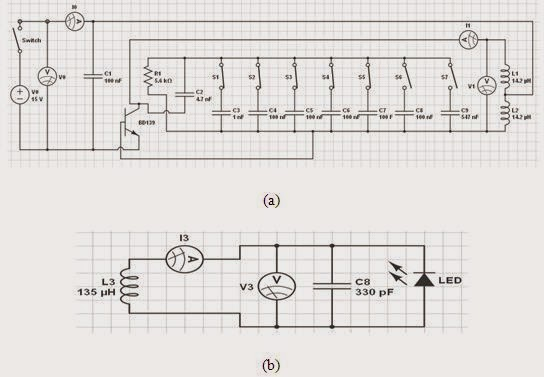Figure 4.7 : (a) Transmitter circuit 774 KHz (b) Receiver signal form 754 KHz

The magnitude of the current and voltage values after measurement is as follows.Table 4.6 Measurement results of 725 KHz Oscillator Frequency

#### 4.3.6 Result of Measurement and Analysis of Wireless Power Transmission System of Air Core Receiver Coil With Frequency 711 KHz

To obtain a frequency value of 711 KHz, the capacitor C2-C8 in the circuit Figure below is in the on condition (switch close). For more details can be seen in the following image. While on the receiving side, the provisions are in accordance with those described in the previous sub-chapter.Figure 4.8 : (a) Transmitter circuit 711 KHz (b) Receiver signal form 715 KHz

The magnitude of the current and voltage values after measurements are made is as follows:Table 4.7 Measurement results of 711 KHz Oscillator Frequency

#### 4.3.7 Result of Measurement and Analysis of Wireless Power Transmission System of Air Core Receiving Coil With 650 KHz Frequency

To obtain a frequency value of 650 KHz, capacitors C2-C9 in the circuit Figure below are in the on condition (switch close). For more details can be seen in the following image. While on the receiving side, the provisions are in accordance with those described in the previous sub-chapter.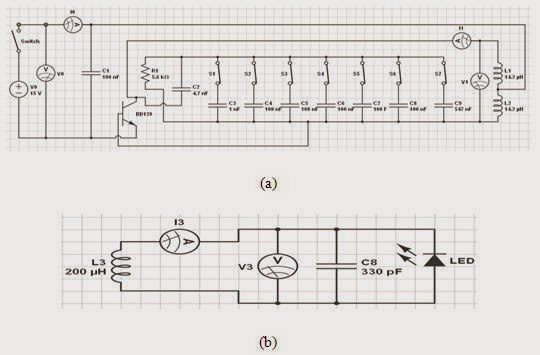Figure 4.9 : (a) Transmitter circuit 650 KHz (b) Receiver signal form 619 KHz

The magnitude of the current and voltage values after measurement is as follows.Table 4.8 Measurement results of the 650 KHz oscillator frequency

Based on the measurement data above, it can be seen that:

1. The greater the distance between the sender and the receiver, it turns out that the power received in the receiver circuit is getting smaller, which also means that the power efficiency will decrease as the distance between the sender and receiver increases.
2. There is a certain frequency range over which the WPT can transmit power with maximum efficiency in this case at 774 KHz. If the frequency is lowered or increased from that frequency, the power delivery will decrease.

As proof, the following is the efficiency data for each frequency at a fixed distance of 2 cm.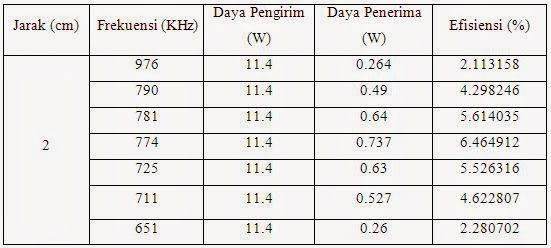Table 4.9 Efficiency Comparison at a fixed distance of 2 cm

If you look at the data in Table 4.4 above, there is a symmetrical relationship between each frequency on power and delivery efficiency, which if depicted in graphical form is as follows.Figure 4.10 Graph of the Relationship Between Frequency and Power Delivery Efficiency

From the data in the table and graph above, it can be seen that at a frequency of 774 KHz, the efficiency of power delivery in the WPT system reaches its maximum value. This is because at that frequency, the impedance in the oscillator circuit reaches a minimum value so that the current that can flow in the sending coil is maximum.

For more details regarding the relationship between distance and power and frequency of this Wireless Electrical Energy Transfer system, can be seen in the following graph.Figure 4.11 Graph of the Relationship Between Distance and Power

From the graph above, it can be seen that the largest power delivery is at a frequency of 744 KHz. This is because the value of the inductive reactance (and capacitive reactance) in the oscillator circuit is the same (minimum impedance) so that the current flowing in the sending coil is maximized, which results in the magnitude of the electromagnetic field emitted by the sending coil to the receiver.

### The relationship between distance and delivery efficiencyAs with power, the highest efficiency is found at a frequency of 774 KHz. This is because the electromagnetic field emitted at this frequency is greater than at other frequencies.